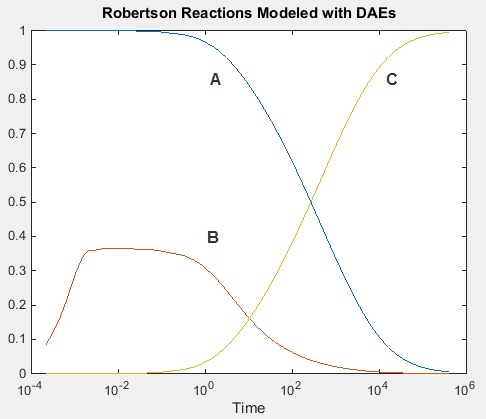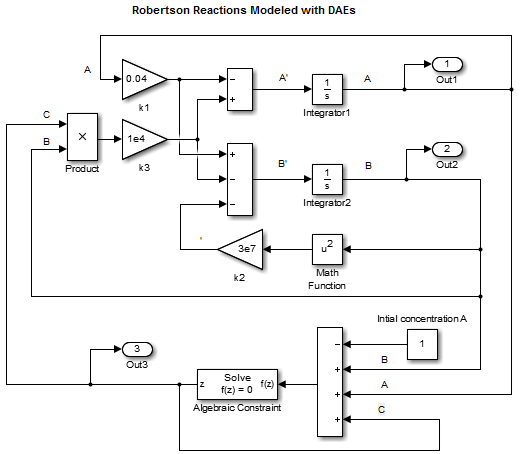Documentation

Model Differential Algebraic Equations

Overview of Robertson Reaction Example

Robertson  created a system of autocatalytic chemical reactions to test and compare numerical solvers for stiff systems. The reactions, rate constants (k), and reaction rates (V) for the system are given as follows:

$\begin{array}{ccccc}A& \stackrel{{k}_{1}}{\to }& B& {k}_{1}=0.04& {V}_{1}={k}_{1}\left[A\right]\\ B+B& \stackrel{{k}_{2}}{\to }& C+B& {k}_{2}=3\cdot {10}^{7}& {V}_{2}={k}_{2}\left[B\right]\left[B\right]\\ B+C& \stackrel{{k}_{3}}{\to }& A+C& {k}_{3}=1\cdot {10}^{4}& {V}_{3}={k}_{3}\left[B\right]\left[C\right]\end{array}$

Because there are large differences between the reaction rates, the numerical solvers see the differential equations as stiff. For stiff differential equations, some numerical solvers cannot converge on a solution unless the step size is extremely small. If the step size is extremely small, the simulation time can be unacceptably long. In this case, you need to use a numerical solver designed to solve stiff equations.

Simulink Model from ODE Equations

A system of ordinary differential equations (ODE) has the following characteristics:

• All of the equations are ordinary differential equations.

• Each equation is the derivative of a dependent variable with respect to one independent variable, usually time.

• The number of equations is equal to the number of dependent variables in the system.

Using the reaction rates, you can create a set of differential equations describing the rate of change for each chemical species. Since there are three species, there are three differential equations in the mathematical model.

$\begin{array}{l}{A}^{\prime }=-0.04A+1\cdot {10}^{4}BC\\ {B}^{\prime }=0.04A-1\cdot {10}^{4}BC-3\cdot {10}^{7}{B}^{2}\\ {C}^{\prime }=3\cdot {10}^{7}{B}^{2}\end{array}$

Initial conditions: $A=1$, $B=0$, and $C=0$.

Build the Model

Create a model, or open the model ex_hb1ode.

1. Add three Integrator blocks to your model. Label the inputs A', B', and C', and the outputs A, B, and C respectively.

2. Add Sum, Product, and Gain blocks to solve each differential variable. For example, to model the signal C',

1. Add a Math Function block and connect the input to signal B. Set the Function parameter to square.

2. Connect the output from the Math Function block to a Gain block. Set the Gain parameter to 3e7.

3. Continue to add the remaining differential equation terms to your model.

3. Model the initial condition of A by setting the Initial condition parameter for the A Integrator block to 1.

4. Add Out blocks to save the signals A, B, and C to the MATLAB variable yout.Simulate the Model

Create a script that uses the sim command to simulate your model. This script saves the simulation results in the MATLAB variable yout. Since the simulation has a long time interval and B initially changes very fast, plotting values on a logarithmic scale helps to visually compare the results. Also, since the value of B is small relative to the values of A and C, multiply B by $1\cdot {10}^{4}$ before plotting the values.

1. Enter the following statements in a MATLAB® script. If you built your own model, replace ex_hblode with the name of your model.

sim('ex_hb1ode')
yout(:,2) = 1e4*yout(:,2);
figure;
semilogx(tout,yout);
xlabel('Time');
ylabel('Concentration');
title('Robertson Reactions Modeled with ODEs')
2. From the Simulink® Editor, on the Modeling tab, click Model Settings.

— In the Solver pane, set the Stop time to 4e5 and the Solver to ode15s (stiff/NDF).

— In the Data Import pane, select the Time and Output check boxes.

3. Run the script. Observe that all of A is converted to C.Simulink Model from DAE Equations

A system of differential algebraic equations (DAE) has the following characteristics:

• It contains both ordinary differential equations and algebraic equations. Algebraic equations do not have any derivatives.

• Only some of the equations are differential equations defining the derivatives of some of the dependent variables. The other dependent variables are defined with algebraic equations.

• The number of equations is equal to the number of dependent variables in the system.

Some systems contain constraints due to conservation laws, such as conservation of mass and energy. If you set the initial concentrations to$A=1$, $B=0$, and $C=0$, the total concentration of the three species is always equal to $1$ since $A+B+C=1$. You can replace the differential equation for ${C}^{\prime }$with the following algebraic equation to create a set of differential algebraic equations (DAEs).

$C=1-A-B$

The differential variables A and B uniquely determine the algebraic variable C.

$\begin{array}{l}{A}^{\prime }=-0.04A+1\cdot {10}^{4}BC\\ {B}^{\prime }=0.04A-1\cdot {10}^{4}BC-3\cdot {10}^{7}{B}^{2}\\ C=1-A-B\end{array}$

Initial conditions: $A=1$and $B=0$.

Build the Model

Make these changes to your model or to the model ex_hb1ode, or open the model ex_hb1dae.

1. Delete the Integrator block for calculating C.

2. Add a Sum block and set the List of signs parameter to +– –.

3. Connect the signals A and B to the minus inputs of the Sum block.

4. Model the initial concentration of A with a Constant block connected to the plus input of the Sum block. Set the Constant value parameter to 1.

5. Connect the output of the Sum block to the branched line connected to the Product and Out blocks.Simulate the Model

Create a script that uses the sim command to simulate your model.

1. Enter the following statements in a MATLAB script. If you built your own model, replace ex_hbldae with the name of your model.

sim('ex_hb1dae')
yout(:,2) = 1e4*yout(:,2);
figure;
semilogx(tout,yout);
xlabel('Time');
ylabel('Concentration');
title('Robertson Reactions Modeled with DAEs')
2. From the Simulink Editor, on the Modeling tab, click Model Settings.

— In the Solver pane, set the Stop time to 4e5 and the Solver to ode15s (stiff/NDF).

— In the Data Import pane, select the Time and Output check boxes.

3. Run the script. The simulation results when you use an algebraic equation are the same as for the model simulation using only differential equations.Simulink Model from DAE Equations Using Algebraic Constraint Block

Some systems contain constraints due to conservation laws, such as conservation of mass and energy. If you set the initial concentrations to$A=1$, $B=0$, and $C=0$, the total concentration of the three species is always equal to $1$ since $A+B+C=1$.

You can replace the differential equation for ${C}^{\prime }$with an algebraic equation modeled using an Algebraic Constraint block and a Sum block. The Algebraic Constraint block constrains its input signal F(z) to zero and outputs an algebraic state z. In other words, the block output is a value needed to produce a zero at the input. Use the following algebraic equation for input to the block.

$0=A+B+C-1$

The differential variables A and B uniquely determine the algebraic variable C.

$\begin{array}{l}{A}^{\prime }=-0.04A+1\cdot {10}^{4}BC\\ {B}^{\prime }=0.04A-1\cdot {10}^{4}BC-3\cdot {10}^{7}{B}^{2}\\ C=1-A-B\end{array}$

Initial conditions: $A=1$, $B=0$, and $C=1\cdot {10}^{-3}$.

Build the Model

Make these changes to your model or to the model ex_hb1ode, or open the model ex_hb1dae_acb.

1. Delete the Integrator block for calculating C.

2. Add an Algebraic Constraint block. Set the Initial guess parameter to 1e-3.

3. Add a Sum block. Set the List of signs parameter to –+++.

4. Connect the signals A and B to plus inputs of the Sum block.

5. Model the initial concentration of A with a Constant block connected to the minus input of the Sum block. Set the Constant value parameter to 1.

6. Connect the output of the Algebraic Constraint block to the branched line connected to the Product and Out block inputs.

7. Create a branch line from the output of the Algebraic Constraint block to the final plus input of the Sum block.Simulate the Model

Create a script that uses the sim command to simulate your model.

1. Enter the following statements in a MATLAB script. If you built your own model, replace ex_hbl_acb with the name of your model.

sim('ex_hb1dae_acb')
yout(:,2) = 1e4*yout(:,2);
figure;
semilogx(tout,yout);
xlabel('Time');
ylabel('Concentration');
title('Robertson Reactions Modeled with DAEs and Algebraic Constraint Block')
2. From the Simulink Editor, on the Modeling tab, click Model Settings.

— In the Solver pane, set the Stop time to 4e5 and the Solver to ode15s (stiff/NDF).

— In the Data Import pane, select the Time and Output check boxes.

3. Run the script. The simulation results when you use an Algebraic Constraint block are the same as for the model simulation using only differential equations.Using an Algebraic Constraint block creates an algebraic loop in a model, If you set the Algebraic Loop parameter to warning (on the Modeling tab, click Model Settings, then select Diagnostics), the following message displays in the Diagnostic Viewer during simulation.For this model, the algebraic loop solver was able to find a solution for the simulation, but algebraic loops don’t always have a solution, and they are not supported for code generation. For more information about algebraic loops in Simulink models and how to remove them, see Algebraic Loop Concepts.

 Robertson, H. H. “The solution of a set of reaction rate equations.” Numerical Analysis: An Introduction (J. Walsh ed.). London, England:Academic Press, 1966, pp. 178–182.